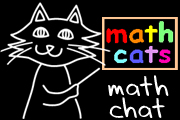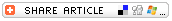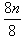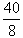## Search form# Math MagicMath magic tricks can liven up any math class and create a sense of wonder and curiosity about math. Not only that, math magic creates a new context for algebraic reasoning as students go beyond "What's the answer?" to explore "What's the trick?"

## TRY A FEW TRICKSAbracadabra! Your number is 13! Images courtesy of Wendy Petti.

Operate on a starting number and always end up with a given number.

One type of number trick involves adding, subtracting, multiplying, or dividing by a starting number and subtracting the original number in such a way that each participant always arrives at a certain number:

Think of a number between 1 and 100.
Multiply this number by 2.
Divide this number by 8.

Think of a number between 1 and 100.
Multiply this number by 3.
Divide this number by 6.

Rearrange digits.

Another type of number trick involves rearranging and recombining digits:

Write a three-digit number (with three different digits).
Mix up the digits to get another three-digit number.
Subtract the smaller number from the larger.
Add the digits in the difference. (If you get a two-digit answer, add the two digits to get a single digit.)
Subtract 5 to get a final number.

(This trick works with numbers up to eight digits, when each digit is different.)

Write a three-digit number. The first and last digits should have a difference of more than one.
Reverse the digits and write down this new number.
Subtract the smaller number from the larger one.
Reverse the digits and add this new number to the circled number.

The starting number appears in a final custom number.

Some number tricks come back to a form of the starting number(s):

Here is a magic number: 12,345,679 (Write it on the board.)
Pick a number from 1 to 9.
Multiply the magic number by your new number.

Surprise! Look at the number you have now!
(It's a string of nine digits made up entirely of each person's starting number. When starting with 2, the new number is 222,222,222.)4

Write the year of your birth.
Double it.
Multiply by 50.
Subtract 615.

The first four digits are the year of your birth. The last two digits are your age.5

## OTHER TYPES OF MATH MAGIC

You can find math magic inWhat do you get when you cut a Mobius strip in half?Â  (a longer Mobius strip!)
• calendars;
• a deck of cards;
• handmade cards with specially created sets of numbers;
• everyday objects: Introduce students to a realm of mathematics they might never have heard of -- topology -- as they ooh and ahh over paper clips leaping and linking together when a dollar bill is snapped, or over the wonders of a Mobius strip.

The resources at the end of this article encompass a variety of math magic ideas presented in kid-friendly fashion.

## Â

Add to the fun and sense of wonder by throwing in some dramatic effects:
• Wear a magician's hat and cape, wave a magic wand, listen to a puppet friend whisper in your ear, or gaze into a crystal ball as you announce the answer in a dramatic voice.
• Before beginning a trick the answer to which you know in advance, write the answer on a piece of paper, fold it several times, ask a student to keep it in his or her pocket "so the magic won't escape" and then, after everyone has followed the steps, ask the student to unfold and read the answer.
• Write the answer on a piece of paper ahead of time, place it in a decorated box, and wave your magic wand over it to help the magic number fill the room and enter every person in the room.
• For tricks with one-digit answers, announce that you will hold the answer in pennies in your hand, and do so, without letting students see what youre picking up. At the end of the steps, open your hand to reveal the answer.
• For a variation on a trick whose answer is an odd number, announce that you will hold half of the answer in your hand. Prepare a penny ahead of time by bending it back and forth with two pairs of pliers until it breaks, or by cutting it in half with metal shears, so you can hold 2 or 3 or 4 pennies in your hand.6
• Select a student assistant. Instead of asking the students to double a number or multiply by two, ask the assistant to close his or her eyes and concentrate hard. Say, "______ [name of assistant] is sending you the same number in your mind. Add it to your first number."
• Work with some of your students ahead of time so they can put on a math magic show for their classmates or another class, or invite one student each day to present a new trick.Wendy Petti is the creator of the award-winning Math Cats Web site, author of Exploring Math with MicroWorlds EX (LCSI, 2005), and a frequent presenter at regional and national math and technology conferences. She teaches grade 4 math at Washington International School.

Adapt number tricks for younger students by asking them to choose a starting number less than 20 or even less than 10.

Provide calculators as needed for multiplying and dividing, or for double-checking answers.

After enjoying a math magic show, guide students in understanding how one of the tricks works. See "How Did You Do That?" below.

Challenge older or advanced students to figure out on their own how a trick works and then explain it so everyone else can understand. Students could work in small groups to explain a trick quickly and effectively.

Ask students to develop their own math magic tricks.

## BUILT-IN BENEFITS

Many math magic tricks call on students to compute with the four basic operations -- sometimes applied to very large numbers. In the context of math magic, computational practice is fun.

If a trick works for some students and not others, it's amazing how eager they can be to find and fix their mistakes so the trick will come out right.

Best of all, many students have an inner motivation to understand how math magic tricks work, and that curiosity can lead them to embrace and apply both new and familiar concepts and skills, including algebra.

## HOW DID YOU DO THAT?

For the most part, the resources cited in this article do not explain their tricks, because "Magicians never reveal their secrets!" You might choose for the tricks to stay in the realm of magic, to intrigue and delight your students. There can be value enough in students' enthusiastic manipulation of numbers as they are being wowed. But there can be value, as well, in revealing a few magicians' secrets.

Most math magic tricks can be understood with algebraic reasoning. Students as young as fourth grade can grasp how simpler tricks work if you guide them step by step through the reasoning and explain the algebraic notation. For example, the first trick can be explained like this:

 Think of a number between 1 and 100. Let's represent this number as n. Multiply your number by 4. We can show this as 4n (4 times n). Add 12. 4n + 12 Multiply this number by 2. 2 (4n + 12) = 8n + 24 (using the Distributive Property) Add 16. (8n + 24) + 16 = 8n + (24 + 16) = 8n + 40 (using the Associative Property) Divide this number by 8.=+= n + 5 Subtract your original number. n + 5 â n = (n â n) + 5 = 0 + 5 (using the Commutative and Associative Properties) Your number is 5! 0 + 5 = 5

It's a wonderful feeling when students beg to know how a trick works and exclaim over the algebraic reasoning that proves it. Demystifying a math magic trick can help students appreciate the greater "magic" and utility of algebra, number properties, and patterns, driven by their own need to know.

But don't overdo it! There's a lot to be said for retaining some of the mystery too, and letting students enjoy the wonder of the "magic."

Notes: 1 This trick is from Mathemagic.
2, 4, 5 These tricks are from Arithmetricks.
3 This trick comes from The I Hate Mathematics! Book.
6 This idea comes from Mathemagic.